## Connecting the Primes to the Logarithmic Integral with Linnik's Identity

Quick Summary: This article will try to show, at a high level, one connection between the logarithmic integral and the prime counting function, using a bit of real valued calculus and some combinatorial properties. No complex analysis or numbers will be needed to follow the argument.

Quite some time ago, the great mathematician Carl Friedrich Gauss speculated that a certain function, the logarithmic integral, is a strikingly good approximation for the prime number counting function. If you're the sort of person for whom math inspires curiousity rather than dread, you might find this fact intriguing. And it is intriguing!

A bit later, in the middle of the 19th century, Bernhard Riemann provided an explicit way to connect the number of primes to the logarithmic integral. This introduced the world to the Riemann Zeta function and its perplexing zeroes.

Riemann's work is amazing, powerful, and fascinating. It's also really challenging. Just following the basic argument that connects the prime counting function to the logarithmic integral requires quite a lot of comfort with complex analysis.

Maybe you're one of those people who finds the logarithmic integral-prime numbers connection fascinating, but has struggled to work through Riemann's complex mathematical machinery, or wouldn't even know where to begin.

If you are, I'm going to try to do something for you here. Using a certain combinatorial identity, I'm going to try to show a connection between the distribution of prime numbers and the logarithmic integral. There will be no complex analysis or even complex numbers. There will only be a fairly light dusting of calculus, and a fair bit of combinatorial work. My argument will be by no means rigorous, but it will be moderately interactive.

Sound interesting? Here we go!

Linnik's Identity

We're going to begin with an awfully handy and powerful combinatorial identity. It is an identity discovered by Yuri Linnik in the middle of the 20th century. It says that, if you know how many ways a number can be expressed as certain combinations of smaller numbers multiplied together, you can tell if it is prime or not.

That might sound silly (after all, if a number can be expressed as any smaller non-1 numbers multiplied together, it's obviously not prime, right?), but it is in fact astonishingly useful.

If you have Flash installed, you can try playing with the identity here in your browser. Make sure to keep your mouse hovering over the app for it to run.

So that's Linnik's identity. Make sure to play around with different numbers to get a sense of how it works.

Sadly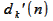is generally a huge nuisance to calculate and reason about, so at first blush this seems like a dubious identity.

Summing Linnik's Identity

One trick to make Linnik's Identity extremely powerful, though, and to avoid factoring individual numbers, is to use it in bulk.

If we apply Linnik's Identity to a range of numbers, from 1 all the way up to some number n, the task of working with the the count of divisor functions changes pretty radically.

So let's take a look at what that sum looks like.

It's a little harder to see what's going on here, I'm afraid. The calculation on the bottom right is Linnik's identity summed from 1 to n. The sum on the bottom left is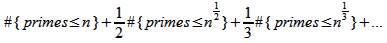which we're going to refer to as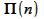. It might not seem obvious, but there's a pretty straightforward way to get just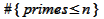from. Riemann himself gave his formula as connectingto the Logarithmic Integral, so that's just what nature seems to intend. So, that's the value we're going to be really interested in.

(Just in case you're curious,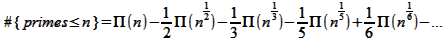At any rate, here's what I hope you can see so far. If we can say interesting things about these sums that I'm calling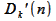, then we might be able to say some interesting things about. If we can find ways to countquickly, we will be able to countquickly too (and, in turn,).

Here is one of the most straightforward ways to express the first few of these – not fast or clever, but it'll get the job done: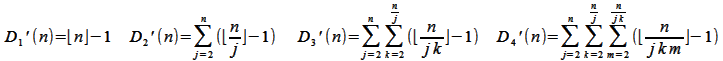I'm not going to say more about counting thefunctions, but if you find this interesting, you might want to take a look here. You can find my fastest Linnik's identity-based prime counting algorithm, which operates in O(n^2/3 ln n) time and O( n^1/3 ln n) space, here.

Approximating the Count of Divisor Sums

As the link at the end of the last section gestures at, trying to get exact values for thefunctions is an interesting but vexing challenge. A pretty natural question to ask is, are there any easier ways to approximate these functions?

Let's start with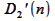, which we can restate as the answer to this question: how many pairs of whole numbers satisfy the constraints,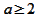, and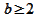?

One obvious approach is to relax the rule about whole numbers – real number functions are often much, much easier to approximate. So we can turn to a bit of calculus and we can ask, instead, what is the area under the curve bounded by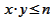? As for the other two constraints, we're going to think of the whole number solutions as squares running from (a-1,b-1) to (a,b) and so occupying 1 full unit of area. To account for this, for real numbers, we're going to have the constraints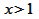, and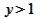.

So, we can say that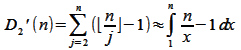( starting the integral at 1 accounts for, and the -1 accounts for). Working through this integral leaves us with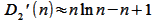That looks like this:

Let's move on to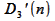, which we can restate as: how many triplets of whole numbers satisfy the constraints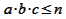,,, and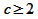?

Once again, we're going to ask about the volume under the real number curve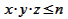, where,, and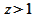. And so we're saying, essentially (with the -1 accounting for),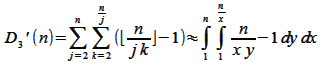which we can evaluate as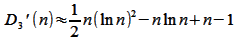It looks like this:

We can do the same thing for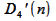, which will give us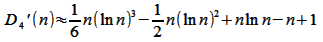Hopefully you can see the bigger pattern here. If we have, one way to approximate it is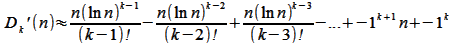I'm going to skip the question of how good these approximations are; the only thing that matters is that you can follow roughly why I'm taking a look at them.

Approximating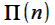So, now we have some approximations for thesums. We know, back from our “Summing Linnik's Identity” section that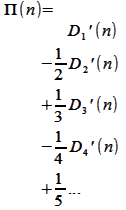So let's try swapping out oursums with their approximations and see what happens.

That means we have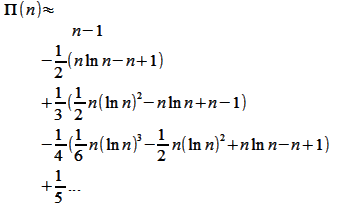which really doesn't look like much of anything.

A funny thing happens if you try to evaluate it, though...

The Logarithmic Integral

The logarithmic integral is defined (fudging over a bit of messiness to avoid a singularity) as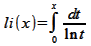If we want to compute it (and we do!), we can turn to the following infinite sum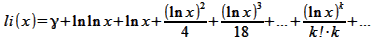For just a second, I want to ignore those first two terms (the first of these is a constant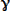, the Euler-Mascheroni constant, and the second term grows really slowly) , and only look at this part of that infinite sum, which is obviously the meat of the logarithmic integral: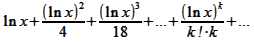Let's compare the output for this series with the output for our approximation ofabove.

You might be inclined to notice that they are identical.

UPDATE(9-13-11) - If you like your arguments in a less empirical form, this identity was proved here by J. M. at math.stackexchange.

Conclusion

So there you have it. Take a summed version of Linnik's identity, swap out the count of divisor sums with the real-valued curves that bound them, and you end up immediately with the Logarithmic Integral. It's a bit hand-wavy, certainly not a rigorous proof in the way that mathematicians tend to care about, but hopefully if you've walked through all this so far, one connection between the prime counting function and the Logarithmic Integral is a bit clearer.

Everything above can be restated in the following tidy way which, to my eye, represents a pretty arresting visual symmetry.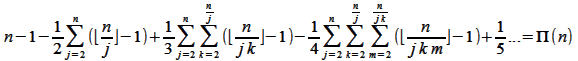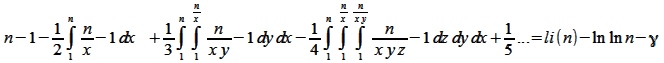Or, which is just another way of rewriting these two identities but might stress the purity of the connection even more,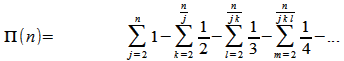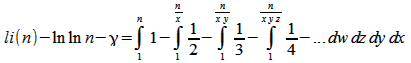From these two identities, it's tempting to say thatis, essentially, a discrete or quantized version of the Logarithmic Integral.is, at least in some sense, the Logarithmic Integral with all the non-integer volume discarded (at least in terms of these identities).

Incidentally, this is part of what makes the Zeroes of the Riemann Zeta Function so significant – they give mathematicians a way to reason about those volumes that have been discarded, amongst other things... but I won't say anymore about that here.

If you find Linnik's Identity interesting (and you should! Its lack of overwhelming force on the internet and in number theory books baffles me!), you might be interested to take a look at some other explorations I've done with the identity. You can use it to count primes pretty quickly , you can invert it to interesting effect, and you can even generalize it.

I have to admit, this was a pretty fun article to put together. If it gets any kind of positive response, I'd love to write up a few more of them.

Nathan McKenzie

9-6-2011

An almost identical approach can be taken for approximating the Mertens function. It is perhaps interesting to see that the fractional / non-integer parts of the approximation must be nearly identical (but opposite) to the integer / lattice parts, because they nearly cancel out, resulting in the comparitively tiny ln n.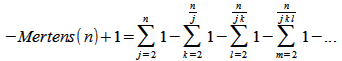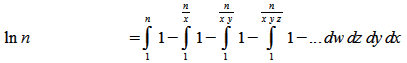A Request

If you find this article intriguing, here's some followon work someone out there can do in exchange, to sate my own curiousity.

I mention, above, in "Approximating the Count of Divisor Sums", that this is just one way to approximate, and thus. There are some other ways to approximate these sums.

Particularly, here, Ivic talks a bit about expressing the principle terms of the divisor summatory function as polynomials made up of ln n to various powers and coefficients constructed from the Stieltjes Constants. (He does this with divisor functions that begins counting at 1, rather than 2, but it is closely related). This is similar to my approach but somewhat different. He then, in turn, refers to a paper by A. F. Lavrik, says this approach can be generalized, and calls it a day.

My own analytical chops aren't yet good enough to follow that generalization, much to my chagrin.

Nevertheless, I am fascinated to know what sort of approximation ofresults from working with these polynomials, rather than from myapproximations above. If anyone finds that an interesting challenge to pursue, I'd love to know the results.